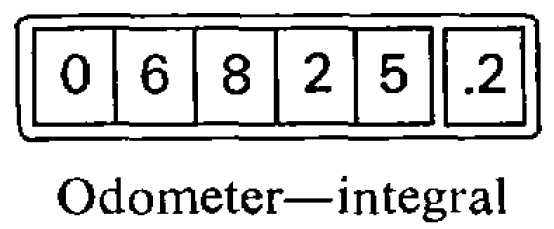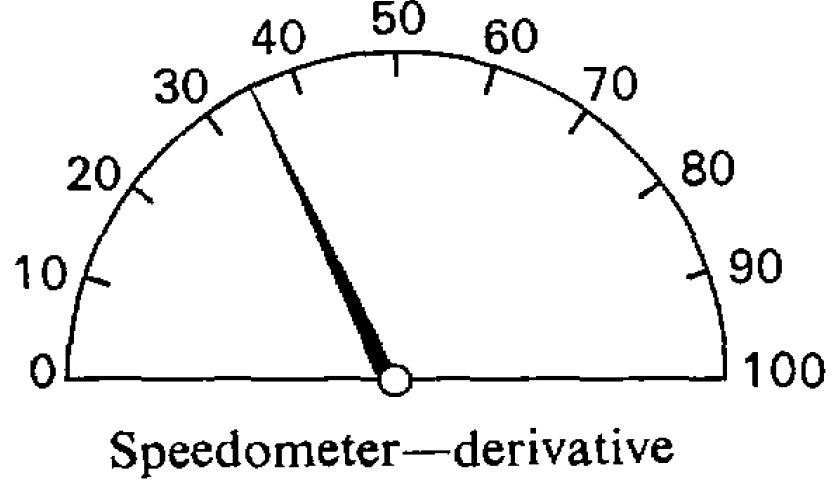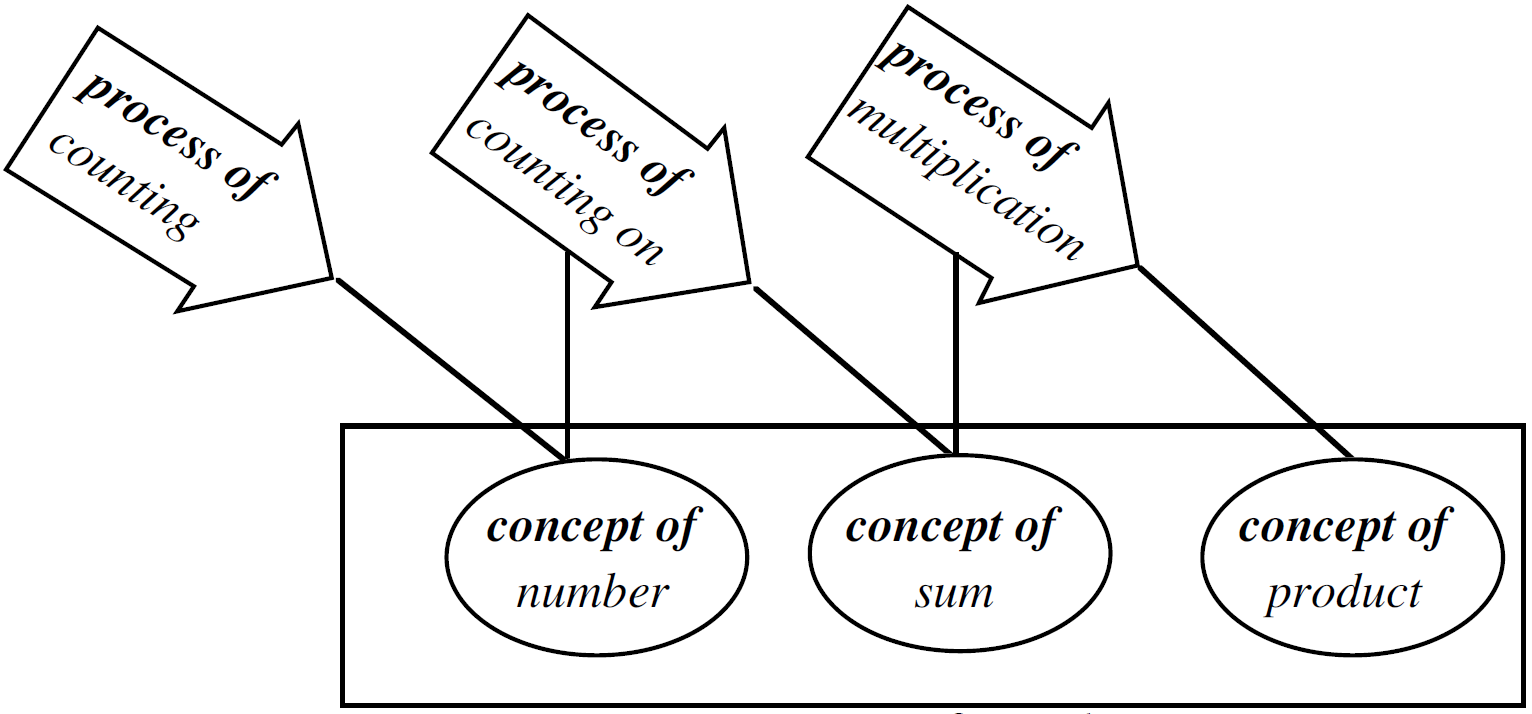## Calculating impact of change for simple models is intuitiveCalculating impact of change of a car 

Calculating impact of change is the second of calculus’s two principal operations

…the two principal symbols that are used in calculating… are:

1. d which merely means “a little bit of.”
Thus dx means a little bit of ..
2. ∫ which is merely a long S, and may be called (if you like) “the sum of.”
Thus ∫ dx means the sum of all the little bits of x… Ordinary mathematicians call this symbol “the integral of.” Now any fool can see that if x is considered as made up of a lot of little bits, each of which is called dx, if you add them all up together you get the sum of all the dx‘s, (which is the same thing as the whole of x). The word “integral” simply means “the whole.” When you see an expression that begins with this terrifying symbol, you will henceforth know that it is put there merely to give you instructions that you are now to perform the operation (if you can) of totalling up all the little bits that are indicated by the symbols that follow. That’s all.

Calculating impact of change means calculating a curve’s footprint

Like every other mathematical operation, the process of differentiation may be reversed; thus, if differentiating y = x4 gives us dy/dx= 4x3; if one begins with dy/dx = 4x3 one would say that reversing the process would yield y = x4. But here comes in a curious point. We should get dy/dx = 4x3 if we had begun with any of the following: x4, or x4 + a, or x4 + c, or x4 with any added constant. So it is clear that in working backwards from dy/dx to y, one must make provision for the possibility of there being an added constant, the value of which will be undetermined until ascertained in some other way.

One use of the integral calculus is to enable us to ascertain the values of areas bounded by curves.

Let AB… be a curve, the equation to which is known. That is, y in this curve is some known function of x. Think of a piece of the curve from the point P to the point Q.

Let a perpendicular PM be dropped from P, and another QN from the point Q. Then call OM = x1 and ON = x2, and the ordinates PM = y1 and QN = y2. We have thus marked out the area PQNM that lies beneath the piece PQ. The problem is, how can we calculate the value of this area?

Calculating impact of change one strip at a time

The secret of solving this problem is to conceive the area as being divided up into a lot of narrow strips, each of them being of the width dx. The smaller we take dx, the more of them there will be between x1 and x2. Now, the whole area is clearly equal to the sum of the areas of all such strips. Our business will then be to discover an expression for the area of any one narrow strip, and to integrate it so as to add together all the strips.

Now think of any one of the strips. It will be like this: being bounded between two vertical sides, with a at bottom dx, and with a slightly curved sloping top.

Suppose we take its average height as being y; then, as its width is dx, its area will be y dx. And seeing that we may take the width as narrow as we please, if we only take it narrow enough its average height will be the same as the height at the middle of it. Now let us call the unknown value of the whole area S, meaning surface. The area of one strip will be simply a bit of the whole area, and may therefore be called dS. So we may write

area of 1 strip = dS = y ∙ dx.

If then we add up all the strips, we get

total area S = ∫ dS = ∫ y dx.

Calculating impact of change from start to finish

…how do you find an integral between limits, when you have got these instructions?

First, find the general integral thus:

∫ y dx,

and, as y = b + ax2 is the equation to the curve…,

∫ (b + ax2) dx

is the general integral which we must find.

Doing the integration in question by the rule…, we get

bx +(a/3)x3 + C;

and this will be the whole area from 0 up to any value of x that we may assign.

Therefore, the larger area up to the superior limit x2 will be

bx2 + (a/3)x23 + C;

and the smaller area up to the inferior limit x1 will be

bx1 + (a/3)x13 + C.

Now, subtract the smaller from the larger, and we get for the area S the value,

area S = b(x2 – x1) + (a/3)( x23 – x13).

This is the answer we wanted.

All integration between limits requires the difference between two values to be thus found. Also note that, in making the subtraction the added constant C has disappeared.

Calculating as simple as possible and no simpler

“Calculus made Easy” shows how… easy most of the operations of the calculus really are. The aim of this book is to enable beginners to learn its language, to acquire familiarity with its endearing simplicities, and to grasp its powerful methods of solving problems…

It can scarcely be denied that the supreme goal of all theory is to make the irreducible basic elements as simple and as few as possible without having to surrender the adequate representation of a single datum of experience.

1. Keisler, H. Jerome. Elementary calculus: An infinitesimal approach. Courier Corporation, 2012, p. xi.
2. Thompson, Silvanus Phillips. Calculus made easy. 2nd ed., enlarged, MacMillan, 1914.
3. Einstein, Albert. “On the method of theoretical physics.” Philosophy of science 1.2 (1934): 163-169.

## Calculating rate of change for simple models is commonsenseCalculating rate of change of a car 

Calculating rate of change is the first of calculus’s two principal operations

…the two principal symbols that are used in calculating… are:

1. d which merely means “a little bit of.”
Thus dx means a little bit of x; or du means a little bit of u.
2. ∫ which is merely a long S, and may be called (if you like) “the sum of.”
Thus ∫ dx means the sum of all the little bits of x… That’s all.

In ordinary algebra which you learned at school, you were always hunting after some unknown quantity which you called x or y; or sometimes there were two unknown quantities to be hunted for simultaneously. You have now to learn to go hunting in a new way; the fox being now neither x nor y. Instead of this you have to hunt for this curious cub called dy/dx. The process of finding the value of dy/dx is called “differentiating.” But, remember, what is wanted is the value of this ratio when both dy and dx are themselves indefinitely small.

Calculating rate of change means calculating a curve’s local slope

It is useful to consider what geometrical meaning can be given to the differential coeffcient. In the first place, any function of x, such, for example, as x2, or √x, or ax + b, can be plotted as a curve…

Consider any point Q on this curve, where the abscissa of the point is x and its ordinate is y. Now observe how y changes when x is varied. If x is made to increase by a small increment dx, to the right, it will be observed that y also (in this particular curve) increases by a small increment dy (because this particular curve happens to be an ascending curve). Then the ratio of dy to dx is a measure of the degree to which the curve is sloping up between the two points Q and T. If… Q and T are so near each other that the small portion QT of the curve is practically straight, then it is true to say that the ratio dy/dx is the slope of the curve along QT.

Calculating rate of change just takes a little algebra and common sense

Now let us see how, on first principles, we can differentiate some simple algebraical expression. Let us begin with the simple expression y = x2. Let x, then, grow a little bit bigger and become x + dx; similarly, y will grow a bit bigger and will become y + dy. Then, clearly, it will still be true that the enlarged y will be equal to the square of the enlarged x. Writing this down, we have:

y + dy = (x + dx)2.

Doing the squaring we get:

y + dy = x2 + 2x∙dx + (dx)2.

Remember that dx meant a bit—a little bit—of x. Then (dx)2 will mean a little bit of a little bit of x; that is… it is a small quantity of the second order of smallness. It may therefore be discarded as quite inconsiderable in comparison with the other terms. Leaving it out, we then have:

y + dy = x2 + 2x∙dx.

Now y = x2; so let us subtract this from the equation and we have left

dy = 2x∙dx.

Dividing across by dx, we find

dy/dx= 2x.

Now this is what we set out to find. The ratio of the growing of y to the growing of x is, in the case before us, found to be 2x.

1. Keisler, H. Jerome. Elementary calculus: An infinitesimal approach. Courier Corporation, 2012, p. xi.
2. Thompson, Silvanus Phillips. Calculus made easy. 2nd ed., enlarged, MacMillan, 1914.

## Math notation serves as pointers to both data and proceduresMath notation works with our limited attention by greatly compressing information

…human brains, though exceedingly complex, are only able to concentrate consciously on a few things at once, requiring a mechanism to cope with the complication:

• “…early processing is largely parallel – a lot of different activities proceed simultaneously. Then there appear to be one or more stages where there is a bottleneck in information processing. Only one (or a few) ‘object(s)’ can be dealt with at a time. This is done by temporarily filtering out the information coming from the unattended objects. The attentional system then moves fairly rapidly to the next object, and so on, so that attention is largely serial (i.e., attending to one object after another) not highly parallel (as it would be if the system attended to many things at once).”
• “Mathematics is amazingly compressible: you may struggle a long time, step by step, to work through some process or idea from several approaches. But once you really understand it and have the mental perspective to see it as a whole, there is often a tremendous mental compression. You can file it away, recall it quickly and completely when you need it, and use it as just one step in some other mental process. The insight that goes with this compression is one of the real joys of mathematics.”

Math notation works by being a pointer to both data and procedures at the same time

…process and concept are combined in a single notion… in the working practices of professional mathematicians and all those who are successful in mathematics. They employ the simple device of using the same notation to represent both a process and the product of that process:

• The symbol 5+4 represents both the process of adding through counting all or counting on and the concept of sum (5+4 is 9).
• The symbol 4×3 stands for the process of repeated addition “four multiplied by three” which must be carried out to produce the product of four and three which is the number 12.
• The algebraic symbol 3x+2 stands both for the process “add three times x and two” and for the product of that process, the expression “3x+2”.

1. Gray, Eddie M., and David O. Tall. “Duality, ambiguity, and flexibility: A ‘proceptual’ view of simple arithmetic.” Journal for Research in Mathematics Education 25.2 (1994): 116-140.
2. Gray, Eddie, and David Tall. “Abstraction as a natural process of mental compression.” Mathematics Education Research Journal 19.2 (2007): 23-40.